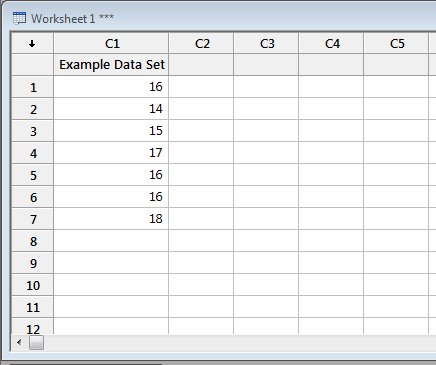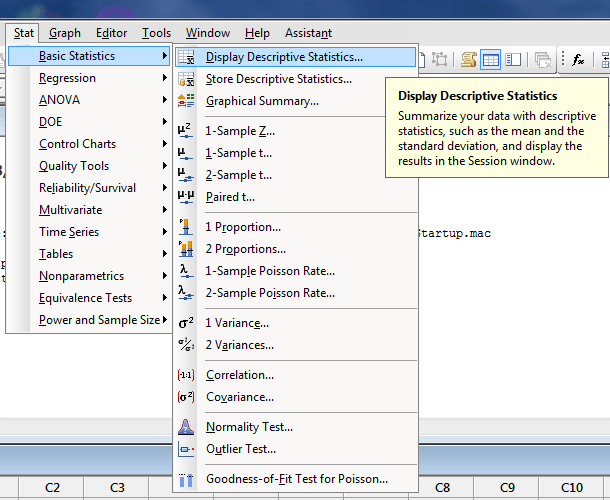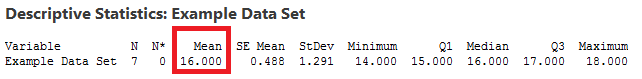﻿ Calculate the mean in minitab - MathBootCamps

# Calculate the mean in minitab

Although it is certainly possible to calculate the mean using the formula (add up the values, divide by how many there are), it is much more efficient and less error prone to use technology. One useful technology tool for calculations and statistics is Minitab. In this article, you will see the steps needed to calculate the mean for any data set in Minitab.

## Step 1: Enter the data into minitab

There is no rule that says you have to use the first column, but if you have only one list of data, go ahead and type it into the column C1. We will use the following data set as an example: 16, 14, 15, 17, 16, 16, 18. Do not enter data in the grayed out space right below C1. Instead, enter it only in the white portion of the spreadsheet.While not required, you should also be sure to label the column/data. To do this, highlight the space right below C1 and type in a name. Then, press enter.## Step 2: Click Stat and select Basic Statistics, then Display Descriptive Statistics…

This is all found at the top menu.Once you click this, a new menu will come up. Here, double click C1 and then click OK.After you click OK, the following will display in the part of the screen above your data. In the figure below, the mean is in the red box.Notice that you also get several other values, such as the number of data values (N), the standard deviation (StDEV), as well as the first and third quartiles (Q1 and Q3).

## Other considerations

If you already have data in Minitab, or you have multiple variables for which you want to calculate the mean, you can simply double click the correct column in step 2 above. Repeating the step for another column, will then show the basic stats, including the mean, for that column as well.## Subscribe to our Newsletter!

We are always posting new free lessons and adding more study guides, calculator guides, and problem packs.

Sign up to get occasional emails (once every couple or three weeks) letting you know what's new!